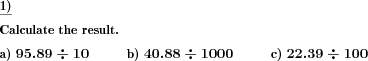Math worksheetsCustom math worksheets at your fingertips

# Details for problem "Decimal fraction div by power of 10"

Quickname: 8600

## Summary

A decimal fraction has to be divided by a power of ten.

## Example## Description

A decimal fraction is presented, it is the dividend in a division problem. The divisor is a power of ten.

The number of places before the decimal point and the number of decimals can be selected for the dividend. For the divisor, the maximum power of ten applied can be defined.

The number of problems can be selected.

Download free worksheets for this math problem here. There is a second sheet with the solutions. Just click on the respective link.Worksheet 1Solution sheetWorksheet 2Solution sheetWorksheet 3Solution sheet

If you can not see the solution sheets for download, they may be filtered out by an ad blocker that you may have installed. If this is the case, please allow ads for this page and reload the page. The solution sheets will then reappear.

• Do the sample worksheets do not really fit?
• Do you need more math worksheets, with a different level of difficulty?
• Would you like to combine different problems on a worksheet and adjust them to your needs?
• As a teacher, you can put together your own worksheets using the automatically generated math problems provided.
With a free initial credit, you can start creating your own math worksheets in a few minutes.

It does not cost anything to try! Register here, to create custom worksheets now!

## Customization options for this problem

ParameterPossible values
Number of problems1, 2, 3, 4, 5, 6, 7, 8, 9, 10
Places0, 1, 2, 3
Decimals places1, 2, 3, 4
Power of 10 up to10, 100, 1000, 10000, 100000, 1000000

Deutsche Version dieser Aufgabe
These informational pages with samples describe math problems that can be combined on custom math worksheets with solutions for home and school use.